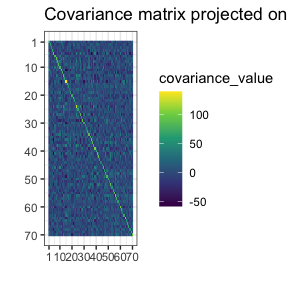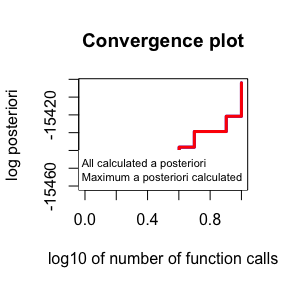# Optimizers

library(gips)
?find_MAP

# What are we optimizing?

The goal of the find_MAP() is to find the permutation $$\sigma$$ that maximizes the a posteriori probability (MAP - Maximum A Posteriori). Such a permutation represents the most plausible symmetry given the data.

This a posteriori probability function is described more in-depth in the Bayesian model selection section of the vignette("Theory"), also available as a pkgdown page. gips can calculate the logarithm of it by log_posteriori_of_gips() function. In the following paragraphs, we will refer to this a posteriori probability function as $$f(\sigma)$$.

# Available optimizers

The space of permutations is enormous - for the permutation of size $$p$$, the space of all permutations is of size $$p!$$ ($$p$$ factorial). Even for $$p=19$$, this space is practically impossible to browse. This is why find_MAP() implements multiple optimizers to choose from:

• "Metropolis_Hastings", "MH"
• "hill_climbing", "HC"
• "brute_force", "BF", "full"

# Metropolis Hastings

This optimizer is implementation of the Second approach from [1, Sec 4.1.2].

This uses the Metropolis-Hastings algorithm to optimize the space; see Wikipedia. This algorithm used in this context is a special case of the Simulated Annealing the reader may be more familiar with; see Wikipedia.

### Short description

In every iteration $$i$$, an algorithm is in a permutation, say, $$\sigma_i$$. Then a random transposition is drawn uniformly $$t_i = (j,k)$$ and the value of $$f(\sigma_i \circ t_i)$$ is computed.

• If new value is bigger than the previous one, (i.e. $$f(\sigma_i \circ t_i) \ge f(\sigma_i)$$), then we set $$\sigma_{i+1} = \sigma_i \circ t_i$$.
• If new value is smaller ($$f(\sigma_i \circ t_i) < f(\sigma_i)$$), then we will choose $$\sigma_{i+1} = \sigma_i \circ t_i$$ with probability $$\frac{f(\sigma_i \circ t_i)}{f(\sigma_i)}$$. Otherwise, we set $$\sigma_{i+1} = \sigma_i$$ with complementary probability $$1 - \frac{f(\sigma_i \circ t_i)}{f(\sigma_i)}$$.

The final value is the best $$\sigma$$ that was ever computed.

### Example

Let’s say we have the data Z from the unknown process:

dim(Z)
#>  50 70
number_of_observations <- nrow(Z) # 50
perm_size <- ncol(Z) # 70
S <- cov(Z) # Assume we have to estimate the mean

g <- gips(S, number_of_observations)
suppressMessages( # message from ggplot2
plot(g, type = "heatmap") +
ggplot2::scale_x_continuous(breaks = c(1,10,20,30,40,50,60,70)) +
ggplot2::scale_y_reverse(breaks = c(1,10,20,30,40,50,60,70))
)g_map <- find_MAP(g, max_iter = 10, optimizer = "Metropolis_Hastings")
#> ========================================================================
#> Warning: The found permutation has n0 = 67 which is bigger than the number_of_observations = 50.
#> ℹ The covariance matrix invariant under the found permutation does not have the likelihood properly defined.
#> ℹ For more in-depth explanation, see 'Project Matrix - Equation (6)' section in vignette('Theory', package = 'gips') or its pkgdown page: https://przechoj.github.io/gips/articles/Theory.html.
g_map
#> The permutation (5,25)(9,45,60)(37,70)
#>  - was found after 10 log_posteriori calculations
#>  - is 15208559032166170453082112 times more likely than the starting, () permutation.

Only after ten iterations the found permutation is unimaginably more likely than the original, () permutation.

plot(g_map, type = "both", logarithmic_x = TRUE)